Journal DetailsFormat
Journal
eISSN
2083-134X
First Published
16 Apr 2011
Publication timeframe
4 times per year
Languages
EnglishOpen Access

# Experimental and theoretical approaches on thermal and structural properties of Zn doped BSCCO glass ceramics

###### Accepted: 21 Dec 2015
Journal DetailsFormat
Journal
eISSN
2083-134X
First Published
16 Apr 2011
Publication timeframe
4 times per year
Languages
English

Thermal properties of Cu–Zn partially substituted Bi1.8Sr2Ca2Cu3.2-xZnxO10+δ (x = 0, 0.1 and 0.5) glass-ceramic systems have been investigated with the help of a differential thermal analyzer (DTA) by using Johnson-Mehl-Avrami-Kolmogorov (JMAK) approximation. Non-isothermal crystallization kinetics of the samples has been tested. The calculated values of activation energy of crystallization (E) and Avrami parameter (n) ranged between 306.1 and 338.3 kJ.mol-1 and 1.29 and 3.59, respectively. Crystallization kinetics was compared following the partial substitution, before and after Zn doping of the sample. In addition, by using a scanning electron microscope (SEM) and X-ray powder diffractometer (XRD), structural properties of Zn doped BSCCO glass-ceramic samples were determined. Surface morphology of the samples was studied by SEM measurements. Lattice parameters and volume of the samples were calculated from the XRD measurements.

#### Keywords

Introduction

Crystallization, providing transformation from glass to glass-ceramics, is obtained by a careful and appropriate thermal process program, which enables nucleation and growth of crystal phases in glass. Ceramics are polycrystalline materials having high mechanical durability and impact resistance, higher refractory and lower thermal expansion coefficient in comparison with glasses from which they are produced . These materials are engineering materials that are used in some special applications because of their hardness, corrosion resistance, oxidation, strength to corrosion and high temperature, dimensional stabilities, optical and other transmission characteristics as well as good electrical properties . Some of these applications include superconductivity, biomedical applications, applications requiring high dielectric constant, material connections and substrates for electronics [5, 6]. Moreover, the glass-ceramic materials are used in aerospace and aviation industry, electronics industry, material coating technology, production of superconducting materials and even in medicine [7, 8]. Glass ceramics, having low thermal expansion and high transparency to the dedicated wavelengths can be used as windows for laser applications .

A lot of additives doped to the BSCCO systems and many features of this system have been investigated. Some of those studies included works with partially substituted Zn–Ca BSCCO systems produced by solid state reaction method . The effect of Zn doping on electrical and magnetic properties of the BSCCO system has been investigated by Mohamed et al. . Aksan et al. analyzed the effect of Zn addition to the BSCCO system prepared by glass-ceramic method in order to investigate the thermal, electrical and magnetic properties . In another study, related to Zn addition, the microhardness properties of the system were investigated . In the literature there are no studies on Zn addition instead of Cu, showing metallic properties of the BSCCO system. Copper is a fundamental element in this structure responsible for its conductivity. For this reason, investigation on Zn–Cu substitution is important. In this study, thermal changes caused by copper deficiency have been analyzed.

In the present work, variation in thermal and structural properties of the glass-ceramic Bi1.8Sr2Ca2Cu3.2-xZnxO10+δ system (x = 0, 0.1 and 0.5) with various Zn doping levels have been studied.

Experimental

One of common methods used for preparing ceramic materials is the glass-ceramic method. Therefore, in this study, the amount of copper in the BSCCO system was reduced by zinc doping using the glass-ceramic method. High purity Bi2O3, SrCO3, CaCO3, CuO and ZnO powders were used as starting materials in the preparation of the samples. 20 g of samples for each doping level (x = 0, 0.1 and 0.5) were prepared. Powders constituting the composition were weighted and mixed in an agate mortar for 1 h to get a homogenous mixture. This final powder was put into an alumina crucible and heated approximately up to 1150 °C for 1.5 h. The molten mixture in the crucible was taken from the furnace and rapidly poured between two copper plates, which were cooled down with liquid nitrogen, then pressed, and as a result, thick black shiny glasses of 1 to 3 mm thickness were obtained. This cold and quick procedure was specially chosen to prevent selective crystallization. Otherwise, partial crystallization might have occurred and as a result, not quite amorphous samples would be obtained. SII7300 Exstar DTA was used to determine the thermal properties of these glass samples at various heating rates (β = 5, 10, 15 and 20 K.min-1) under nitrogen gas atmosphere. After this process, glass-ceramic samples were obtained by sintering the glass samples in a furnace at 840 °C under an oxygen flow for 120 h.

Bruker D8 Advance X-ray powder diffractometer was used to determine the lattice parameters of the samples, with CuKα radiation in the range of 3° ≤2θ≤70° at a scan speed of 2°/min. Phase structure and lattice parameters were obtained by using Bruker-EVA 10.0.1.0 version analyzing program with ICDD PDF2-2002 library. The mean values of lattice parameters of the Bi1.8Sr2Ca2Cu3.2-xZnxO10+δ samples were determined from the high angle (0 0 1) peaks of the XRD measurements. Leo EVO-40 VPX scanning electron microscope was used to determine the surface morphology of the samples. Hereafter, the produced samples were named as CZn00, CZn01 and CZn05 for simplicity.

Theoretical background

As it was indicated by various authors, the isothermal kinetics was firstly derived by Kolmogorov and developed by Johnson, Mehl and Avrami. During the long development history of this process, Erofeev and Mitskevich also gave some contributions . The volume fraction changing in time t is defined as a function of time as:

$x=1−exp[−g∫0tIv((∫t′tudτ)m)dt′]$$$x = 1 - {\rm{exp}}\left[ { - g\int_0^t {{I_v}} \left( {{{\left( {\int_{t'}^t {u{d_\tau }} } \right)}^m}} \right)dt'} \right]$$

Crystallization and reaction kinematics at a constant temperature were expressed by the wellknown Johnson-Mehl-Avrami equation:

$−ln(1−x)=(kt)n$$$- {\rm{ln}}\left( {1 - x} \right) = {\left( {kt} \right)^n}$$

Temperature dependence of k within a specific temperature range can be given by Arrhenius equation:

$k=k0exp(−E/RT)$$$k = {k_0}{\rm{exp}}\left( { - E/RT} \right)$$

At the Differential Thermal Analysis (DTA), heating rate (β = dT/dt) is usually kept constant during scanning. Thus, the reaction rate constant (k), changes with time as the temperature changes. Such cases are referred as non-isothermal conditions. Temperature of a sample and heating rate are linearly dependent on each other as [22, 23]:

$T=T0+βt$$$T = {T_0} + \beta t$$

If we write equation 2in another form, we get:

$x(t)=1−exp[−(kt)n]$$$x\left( t \right) = 1 - {\rm{exp}}\left[ { - {{\left( {kt} \right)}^n}} \right]$$

Time derivative of this equation is:

$x˙=n(kt)n−1(k+kt)(1−x)$$$\dot x = n{\left( {kt} \right)^{n - 1}}\left( {k + kt} \right)\left( {1 - x} \right)$$

For the derivative of k with respect to time, with help of equation 3 and equation 4, equation 7 was obtained:

$k˙=(dkdT)(dTdt)=(βERT2)k$$$\dot k = \left( {{{dk} \over {dT}}} \right)\left( {{{dT} \over {dt}}} \right) = \left( {{{\beta E} \over {R{T^2}}}} \right)k$$

If this equation is substituted in equation 6 we get:

$x˙=nkntn−1(1−at)(1−x)$$$\dot x = n{k^n}{t^{n - 1}}\left( {1 - at} \right)\left( {1 - x} \right)$$

where a = (βE/RT2).

If E/RT2 ≪1, (at) term can be neglected. Thus, equation 8 can be rewritten as :

$x˙=(1−x)nkntn−1$$$\dot x = \left( {1 - x} \right)n{k^n}{t^{n - 1}}$$

If this equation is rearranged according to equation 7 we get:

$x˙=Ank(1−x)$$$\dot x = Ank\left( {1 - x} \right)$$

where A = [ln(1-x)](n-1)/n. If the time derivative of equation 9 is set to zero, by assuming that A is constant at maximum peak temperature (Tx), an equation depending on (Tp) is obtained:

$x¨=Ank(1−x)[(βE/RTx2)−Ank]=0$$$\ddot x = Ank\left( {1 - x} \right)\left[ {\left( {\beta E/RT_x^2} \right) - Ank} \right] = 0$$

This equation can also be written as:

$(β/Tx2)=Cexp(−E/RTx)$$$\left( {\beta /T_x^2} \right) = C{\rm{exp}}\left( { - E/R{T_x}} \right)$$

where C = (ARk0/E). The logarithm of the above equation is:

$ln(β/Tx2)=Sabit−(E/RTx)$$$\ln \left( {\beta /T_x^2} \right) = Sabit - \left( {E/R{T_x}} \right)$$

If the graph of $ln(β/Tx2)$$\ln \left( {\beta /{\rm T}_{\rm x}^2} \right)$ expression is plotted versus 1000/Tx, the slope of this graph gives the fraction of (-E/R) and then the activation energy can be easily calculated. Here, E is the activation energy and R is the gas constant, R = 8.314 J/mol.K. Similarly, k0 value can be determined from $ln(β/Tx2)$$\ln \left( {\beta /{\rm T}_{\rm x}^2} \right)$ versus 1000/Tx graph. k0 can also be found from the intercept of this plot. We can calculate the reaction rate (k) fromequation 3 which is valid for both isothermal and nonisothermal conditions, by substituting the value of the frequency factor in the equation. According to Ozawa method derived from Johnson-Mehl-Avrami-Kolmogorov equation:

$dln[−ln(1−x)]/dlnβ=−n$$$d\ln \left[ { - \ln \left( {1 - x} \right)} \right]/d\ln \beta = - n$$

the slope of ln[-ln(1-x)] versus lnβ graph, plotted according to equation 14, gives the value of −n . To obtain the Avrami parameter (n), which is important in determination of the nucleation mechanism of the samples, the crystallization fraction equation was used :

$x=Ax/A$$$x = {A_x}/A$$

Fig. 1 shows a DTA curve changing with temperature. Here, TStart is the beginning temperature of the peak, Ty is the partial temperature, Tx is the DTA peak temperature, TFinish is the final temperature of the peak, Ax is the partial area of the peak between TStart and Ty, A is the total area (crystallization enthalpy) of the peak between the temperatures TStart and TFinish, and Tg is glass transition temperature.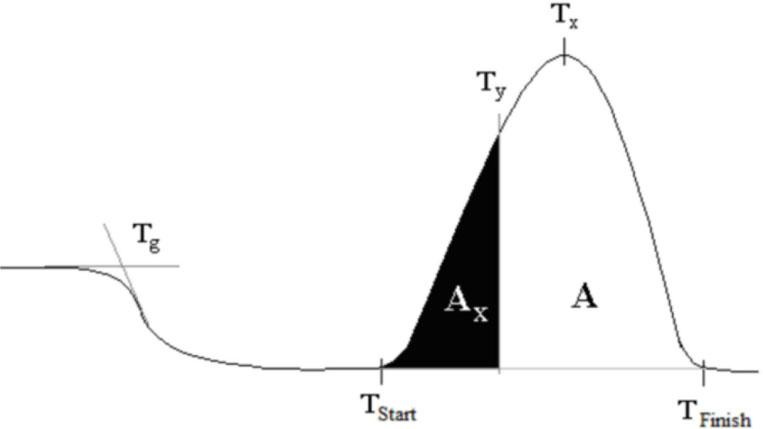DTA curve and the values determined from the graph.

According to Xie and Gao, the Avrami parameters found from surface to inside are interpreted as :

n = 1 - surface nucleation and one dimensional growth,

n = 2 - volume nucleation and one dimensional growth,

n = 3 - volume nucleation and two dimensional growth,

n = 4 - volume nucleation and three dimensional growth.

Glass transition temperatures of the samples were analyzed by using different approaches . The first experimental relation between glass transition temperature and heating rate was given by Lasocka  as:

$Tg=A+Bln(β)$$${T_g} = A + B{\rm{ln}}(\beta )$$

where A and B are constants of a given glass composition.

Results
Thermal properties

Fig. 2 shows the DTA curve of a powder Bi1.8Sr2Ca2Cu2.5Zn0.5O10+δ glass sample at heating rates of 5, 10, 15 and 20 K.min-1. Data gathered from the DTA curves of other samples were listed in Table 1. It is seen from the DTA curves that the crystallization peak temperature intensities increase with increasing heating rate. The crystallization peak temperature variations depending on the system of doping were investigated based on the data from Table 1. As a consequence, it is seen that the glass transition temperature and the crystallization peak temperature are shifted towards higher values with increasing Zn doping. This is because separation of the peaks that are close together becomes harder when the heating rate increases. Glass transition temperature obtained from these values was in the range of 732.0 to 769.0 K. First crystallization peak temperature (Tx1) and second peak temperature (Tx2), corresponding to CuO phase values were obtained in the range of 741.0 to 786.1 K and 796.8 to 819.8 K, respectively. Exothermic peaks, formed around these temperatures, were claimed to occur due to nucleation [29, 30] in the BSCCO system.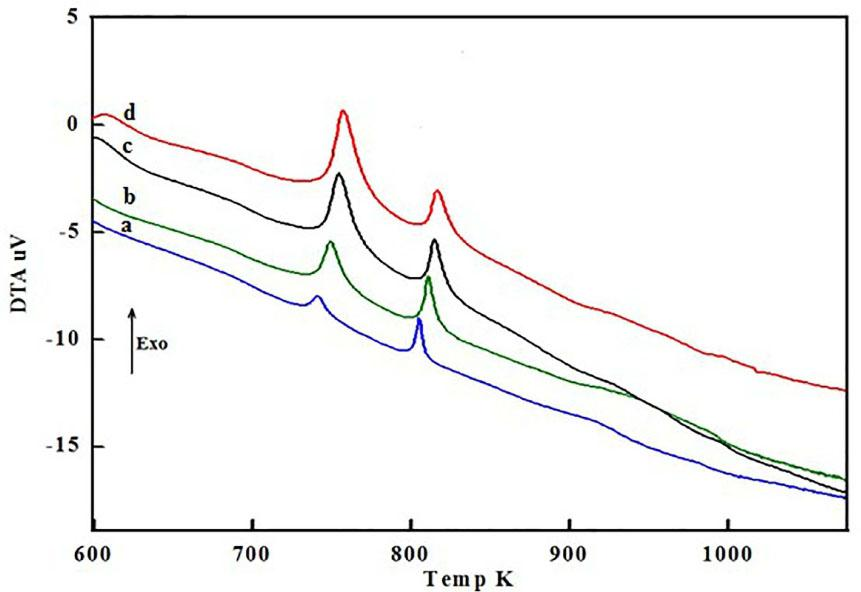DTA curves of Bi1.8Sr2Ca2Cu2.5Zn0.5O10+δ sample for various heating rates (a) β = 5 K.min-1, (b) β =10 K.min-1, (c) β = 15 K.min-1 and (d) β = 20 K.min-1.

DTA results of Bi1.8Sr2Ca2Cu3.2-xZnxO10+δ (x = 0, 0.1, and 0.5) samples.

SamplesHeating Rate (β) [K_min-1]Tg [K]Tx1 [K]Tx2 [K]Lasocka ParametersEn
A [K]B[kJ_mol-1]
CZn 05732.0741.0796.8
10738.0751.4809.0720.47.1338.33.59
15740.0755.7805.0
20742.0759.5808.0
CZn 0.15738.0745.4799.4
10745.0758.2813.0725.87.8312.42.79
15747.0762.0808.3
20749.0765.0812.0
CZn 0.55757.0766.0806.0
10763.0776.3812.4742.49.1306.11.29
15768.0785.0819.2
20769.0786.1819.8

According to the DTA results obtained from Table 1, initial crystallization activation energies were calculated using Kissinger method.

In Fig. 3, the graph of $ln(β/Tx2)$$\ln \left( {\beta /{\rm T}_{\rm x}^2} \right)$ vs. 1000/Tx is plotted for all samples. From the results of calculations obtained by using equation 13, the initial crystallization activation energies of Bi1.8Sr2Ca2Cu3.2-xZnxO10+δ compositions with increasing Zn amount x = 0, 0.1 and 0.5 were found as 338.3, 312.4 and 306.1 kJ.mol-1, respectively. Variation of activation energy depending on the amount of Zn doping showed a decrease with the doping. Crystallization activation energy for the BSCCO system was found between 337 and 367 kJ.mol-1 in the literature . The reason for the decrease in the activation energy is considered as the occurrence of instability in the samples. The decrease in the activation energy in glass-ceramic samples makes them thermodynamically more unstable .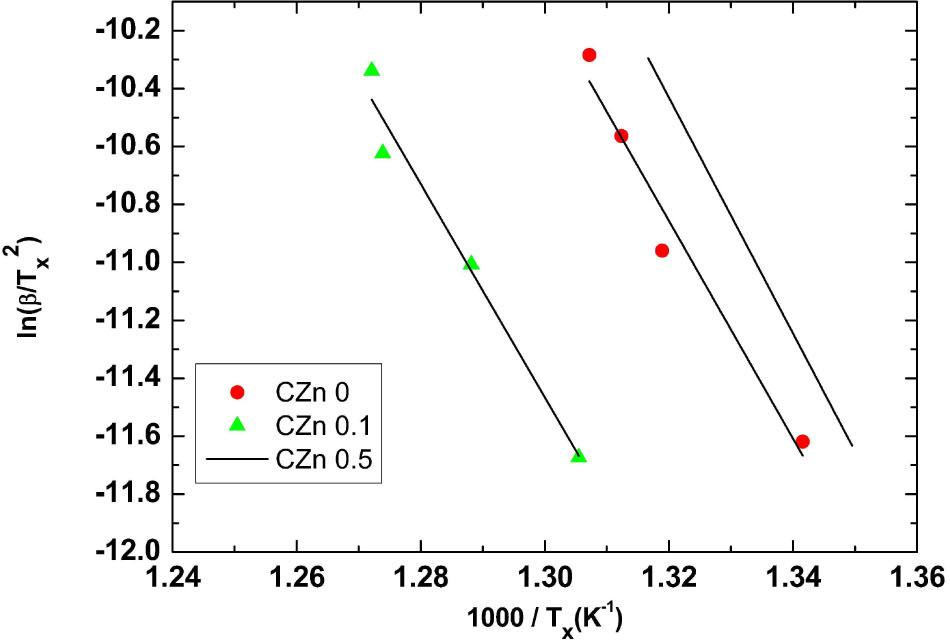ln(β/Tx2)$\ln \left( {\beta /{\rm T}_{\rm x}^2} \right)$ vs. 1000/Tx graph for all samples according to Kissinger formula.

In Fig. 4, Tg versus ln(β) graph was plotted using equation 16 which gives a relation between glass transition temperature and heating rate. A and B parameters in Table 1 were also obtained from this graph. As it is seen from Table 1, the A and B parameters increase with Zn doping. The ln[-ln(1 - x)] versus ln(β) graph drawn with the help of x value calculated from Fig. 2 is show in Fig. 5. The average values of Avrami parameter evaluated from the slope are listed in Table 1. The calculated n values are in the range of 1.29 ≤ n ≤ 3.59, which indicates that the crystallization occurs through different mechanisms.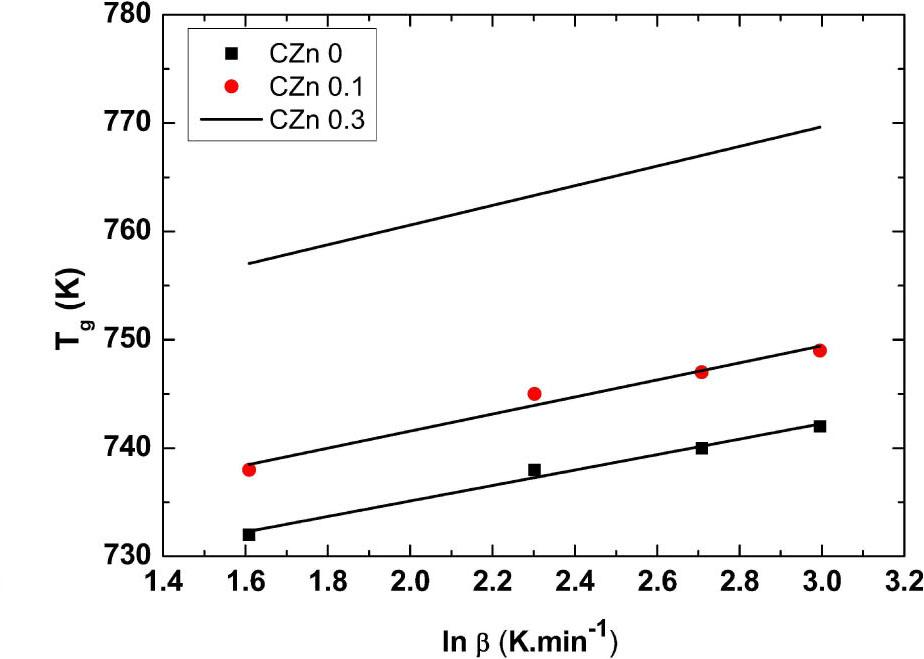Tg versus lnβ(K.min-1) graph of the samples according to Lasocka formula.

Avrami parameter for the reaction is approximately equal to 4. According to this value of n, a volume nucleation and three-dimensional growth occurred. The value of n decreased with the increase in the doping concentration. Therefore, surface nucleation and one-dimensional growth of the sample were confirmed.

Structural properties
XRD analysis

In Fig. 6, the XRD graphs of all glass ceramic samples are given. Bi-2212 (041-0932-ICDD) main and SrO (074-1227 ICDD) impurity phases were determined in the undoped sample.

The peak intensity of the doped samples increases and other new peaks start to appear with the increase in Zn concentration. Some of these peaks are Bi-2201 (046-0040ICDD), CuO (078-0428-ICDD) and CaSrO (048-1471 ICDD) impurity peaks and the others are unidentified impurity peaks.

In Table 2, calculated lattice parameters and volume values are given. The lattice parameters a, b and c were calculated using the d values and (hkl) parameters by assuming orthorhombic symmetry for Bi1.8Sr2Ca2Cu3.2-xZnxO10+δ glassceramic structure, employing the least-squares method and using the data extracted from the XRD measurements. The accuracy in determining the lattice parameters was ±0.005 Å. The lattice parameters a, b and c increase with doping of Zn. The lattice parameter a increases from 5.40 to 5.41 Å and the parameter c increases from 30.78 to 30.82 Å. Related to these parameters, lattice volume increased from 897.55 to 902.34 Å3.

Lattice parameters a, b, c and volume (V) values of all samples.

SamplesV(A˚ 3)a(A˚)b(A˚)c(A˚)
CZn00897.555.405.4030.78
CZn01899.505.415.4030.79
CZn05902.345.415.4130.82
SEM analysis

Surface structure of the samples was examined based on the SEM images (Fig. 7). Crystallization of the sample increased with the increase of Zn doping. Therefore, needle-like and flaky-like grains occured. As can be seen in Fig. 7, the needle like grain morphology of the samples became dominant with doping. The Bi-2223 phase is believed to be the source of the needle-like grains. In all the samples, the needle-like grains are more dominant than the flaky-like grains. According to our previous study, energy dispersive spectroscopy (EDS) results showed that Zn concentration increased with decreasing Cu and Ca concentrations but reduction in Cu dopant was more dominant . Namely, Cu atoms were replaced by Zn atoms in the crystal structure.

Conclusions

In this work, variation in thermal properties of a glass-ceramic Bi1.8Sr2Ca2Cu3.2-xZnxO10+δ system with various Zn doping has been studied with a differential thermal analyzer (DTA) by using Johnson-Mehl-Avrami-Kolmogorov approximation. Non-isothermal crystallization kinetics of the samples has also been investigated. As it is clearly seen from the DTA results, the crystallization peak temperature is shifted to higher temperature values with the increasing heating rate. The reason of this can be explained as follows: the energy required for crystallization at lower heating rates, occurs at lower temperature values. However, the energy required for the nucleation is increased quickly at higher heating rates. Accordingly, the crystallization temperature increases. The decrease in the activation energy of crystallization with increasing Zn doping is caused by the replacement of Cu atoms removed from the crystal structure by Zn atoms incorporated into the structure, and because of the changes in the binding distances of adjacent atoms in the crystal structure. The rearrangement of the atoms in the amorphous structure is possible by exchange of the binding energy with neighboring atoms.

Furthermore, since glass at a stable state allows the doping up to a certain limit, when the amount of Zn doping in the system is increased, thermal activity of the ions in the structure is decreased. For this reason, the quality of the samples in terms of thermal properties decreased with increasing the doping concentration. When the contribution rate of the samples increased, Avrami parameters, which were calculated from crystallization peak areas of DTA curves, decreased. According to the obtained Avrami parameters, surface nucleation occurred as the doping amount increased. The XRD analysis showed that the lattice parameters a, b and c increased with Zn concentration. SEM results showed enhanced crystallization of the sample with increasing amount of Zn doping.DTA curve and the values determined from the graph.DTA curves of Bi1.8Sr2Ca2Cu2.5Zn0.5O10+δ sample for various heating rates (a) β = 5 K.min-1, (b) β =10 K.min-1, (c) β = 15 K.min-1 and (d) β = 20 K.min-1.ln(β/Tx2)$\ln \left( {\beta /{\rm T}_{\rm x}^2} \right)$ vs. 1000/Tx graph for all samples according to Kissinger formula.Tg versus lnβ(K.min-1) graph of the samples according to Lasocka formula.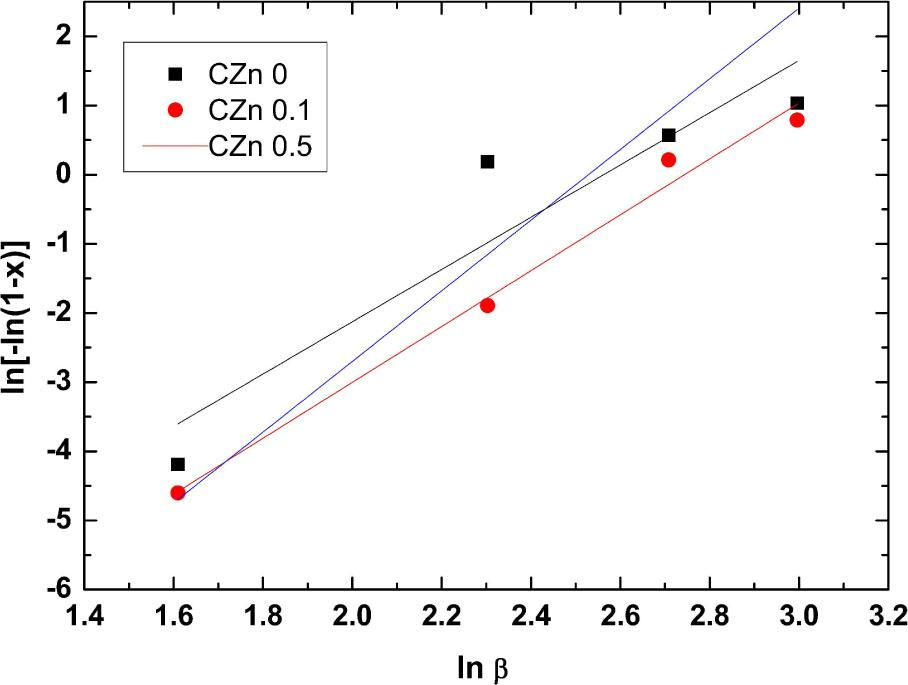ln[-ln(1-x)] versus ln(β) graph for the samples.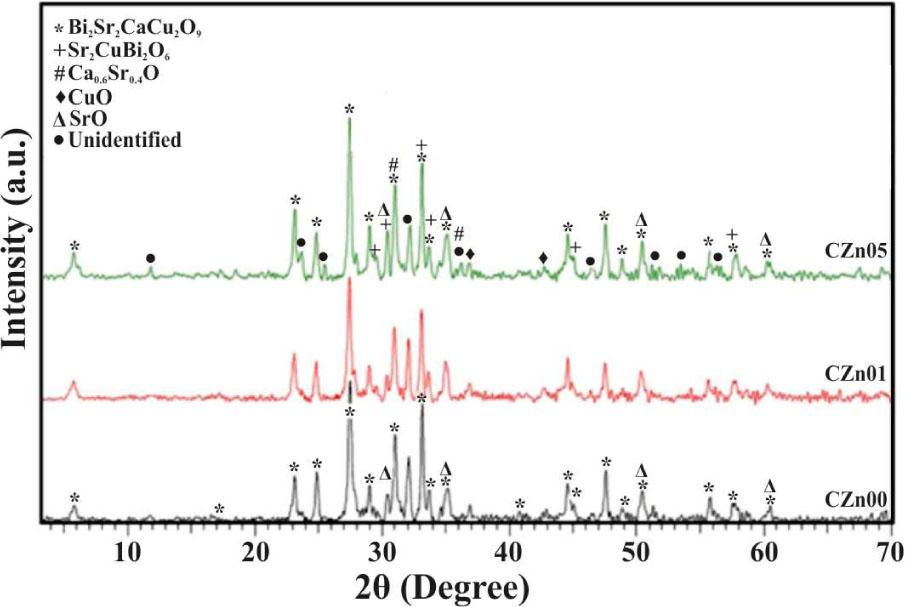XRD patterns of the samples.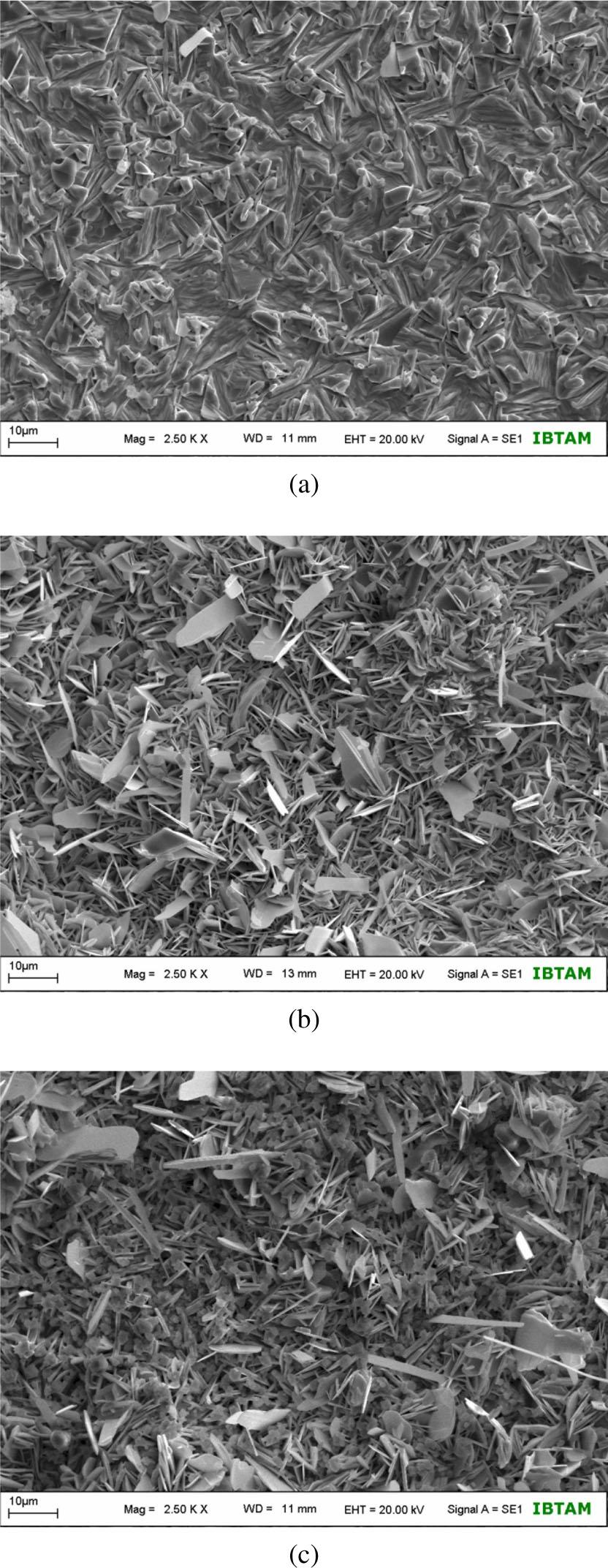SEM micrographs of the (a) CZn00 (b) CZn0.1 and (c) CZn0.5 samples.

#### Lattice parameters a, b, c and volume (V) values of all samples.

SamplesV(A˚ 3)a(A˚)b(A˚)c(A˚)
CZn00897.555.405.4030.78
CZn01899.505.415.4030.79
CZn05902.345.415.4130.82

#### DTA results of Bi1.8Sr2Ca2Cu3.2-xZnxO10+δ (x = 0, 0.1, and 0.5) samples.

SamplesHeating Rate (β) [K_min-1]Tg [K]Tx1 [K]Tx2 [K]Lasocka ParametersEn
A [K]B[kJ_mol-1]
CZn 05732.0741.0796.8
10738.0751.4809.0720.47.1338.33.59
15740.0755.7805.0
20742.0759.5808.0
CZn 0.15738.0745.4799.4
10745.0758.2813.0725.87.8312.42.79
15747.0762.0808.3
20749.0765.0812.0
CZn 0.55757.0766.0806.0
10763.0776.3812.4742.49.1306.11.29
15768.0785.0819.2
20769.0786.1819.8

Askeland D.R., Fulay P.P., The Science and Engineering of Materials, Van Nastrand Reinhold Co. Ltd., Hong Kong, 1988.AskelandDRFulayPPThe Science and Engineering of MaterialsVan Nastrand Reinhold Co. LtdHong Kong1988Search in Google Scholar

Omar A.A., El-Shennawi A.W.A., El Ghannam A.R., J. Mater. Sci., 26 (1991), 6049.OmarAAEl-ShennawiAWAEl GhannamARJ. Mater. Sci261991604910.1007/BF01113882Search in Google Scholar

Mcmillan P.W., Non-Metallic Solids, Glass-Ceramics, Academic Press, London, 1979.McmillanPWNon-Metallic SolidsGlass-Ceramics, Academic PressLondon1979Search in Google Scholar

Holland W., Beall G., Glass-Ceramic Technology, The American Ceramic Society, Ohio, 2002.HollandWBeallGGlass-Ceramic TechnologyThe American Ceramic SocietyOhio2002Search in Google Scholar

Arslan A., Koralay H., Çavdar S., Günen A., J. Non-Cryst. Solids, 358 (2012), 1190.ArslanAKoralayHÇAvdarSGünenAJ. Non-Cryst. Solids3582012119010.1016/j.jnoncrysol.2012.02.016Search in Google Scholar

Çavdar S., Koralay H., Altindal S., J. Low Temp. Phys., 164 (2011), 102.ÇavdarSKoralayHAltindalSJ. Low Temp. Phys164201110210.1007/s10909-011-0361-1Search in Google Scholar

Koralay H., Arslan A., Cavdar S., Ozturk O., Asikuzun E., Gunen A., Tasci A.T., J. Mater. Sci. Mater. Electron., 24 (2013), 4270.KoralayHArslanACavdarSOzturkOAsikuzunEGunenATasciATJ. Mater. Sci. Mater. Electron242013427010.1007/s10854-013-1396-7Search in Google Scholar

Höland W., Rheinberger V., Apel E., Hoen C., Höland M., Dommann A., Obrecht M., Mauth C., Graf-Hausner U., J. Mater. Sci. Mater. Med., 17 (2006), 1037.HölandWRheinbergerVApelEHoenCHölandMDommannAObrechtMMauthCGraf-HausnerUJ. Mater. Sci. Mater. Med172006103710.1007/s10856-006-0441-y17122916Search in Google Scholar

Sanghera J., Villalobos S.B.G., Kim W., Frantz J., Shaw B., Sadowski B., Miklos R., Baker C., Hunt M., Aggarwal I., Kung F., Reicher D., Peplinski S., Ogloza A., Langston P., Lamar C., Varmette P., Dubinskiy M., Desandre L., Opt. Mater., 33 (2011), 511.SangheraJVillalobosSBGKimWFrantzJShawBSadowskiBMiklosRBakerCHuntMAggarwalIKungFReicherDPeplinskiSOglozaALangstonPLamarCVarmettePDubinskiyMDesandreLOpt. Mater33201151110.1016/j.optmat.2010.10.038Search in Google Scholar

Kayed T.S., Calinli N., Aksu E., Koralay H., Günen A., Ercan İ., Aktürk S., C¸ Avdar S., Cryst. Res. Technol., 39 (2004), 1063.KayedTSCalinliNAksuEKoralayHGünenAErcanİAktürkSÇavdarSCryst. Res. Technol392004106310.1002/crat.200410291Search in Google Scholar

Ozturk O., Asikuzun E., Coskunyurek M., Soylu N., Hancerliogullari A., Varilci A., Terzioglu C., J. Mater. Sci.-Mater. El., 25 (2014), 444.OzturkOAsikuzunECoskunyurekMSoyluNHancerliogullariAVarilciATerziogluCJ. Mater. Sci.-Mater. El25201444410.1007/s10854-013-1608-1Search in Google Scholar

Boussouf N., Mosbah M.-F., J. Supercond. Nov. Magn., 26 (2013), 2891.BoussoufNMosbahM-FJ. Supercond. Nov. Magn262013289110.1007/s10948-012-2091-2Search in Google Scholar

Ozturk O., J. Mater. Sci.-Mater. El., 23 (2012), 1235.OzturkOJ. Mater. Sci.-Mater. El232012123510.1007/s10854-011-0580-xSearch in Google Scholar

Mohamed S.B., Halim S.A., Azhan H., Ieee T. Appl. Supercon., 11 (2001), 2864.MohamedSBHalimSAAzhanHIeeeTAppl. Supercon112001286410.1109/77.919659Search in Google Scholar

Aksan M.A., Yakinci M.E., Balci Y., J. Supercond. Nov. Magn., 15 (2002), 6.AksanMAYakinciMEBalciYJ. Supercond. Nov. Magn1520026Search in Google Scholar

Toplu A., Karaca İ., Kölemen U., Ceram. Int., 41 (2015), 953.TopluAKaracaİKölemenUCeram. Int41201595310.1016/j.ceramint.2014.09.014Search in Google Scholar

Johnson W.A., Mehl K.F., Trans. Am. Inst. Min. Met. Eng., 135 (1939), 419.JohnsonWAMehlKFTrans. Am. Inst. Min. Met. Eng1351939419Search in Google Scholar

Avrami M.J., J. Chem. Phys., 7 (1939), 1103.AvramiMJJ. Chem. Phys71939110310.1063/1.1750380Search in Google Scholar

Avrami M.J., J. Chem. Phys., 8 (1940), 212.AvramiMJJ. Chem. Phys8194021210.1063/1.1750631Search in Google Scholar

Erofeev B.V., Mitskevich M., Zh. Fiz. Khim., 24 (1950), 1235.ErofeevBVMitskevichMZh. Fiz. Khim2419501235Search in Google Scholar

Kolmogorov A.N., Izv. Akad. Nauk USSR Ser. Math. Tom., 1 (1937), 225.KolmogorovANIzv. Akad. Nauk USSR Ser. Math. Tom11937225Search in Google Scholar

Vá Squez J., Wagner P., Villares R., Jiménez G., J. Non-Cryst. Solids, 235 (1998), 548.Vá SquezJWagnerPVillaresRJiménezGJ. Non-Cryst. Solids235199854810.1016/S0022-3093(98)00661-9Search in Google Scholar

Gao Y.Q., Wang W., J. Non-Cryst. Solids, 81 (1986), 129.GaoYQWangWJ. Non-Cryst. Solids81198612910.1016/0022-3093(86)90262-0Search in Google Scholar

Kissinger H.E., Anal. Chem., 29 (1957), 1702.KissingerHEAnal. Chem291957170210.1021/ac60131a045Search in Google Scholar

Ozawa T., Polymer, 12 (1971), 150.OzawaTPolymer12197115010.1016/0032-3861(71)90041-3Search in Google Scholar

Xie X., Gao H., J. Non-Cryst. Solids, 240 (1998), 166.XieXGaoHJ. Non-Cryst. Solids240199816610.1016/S0022-3093(98)00673-5Search in Google Scholar

Yousef E., El-Adawy A., Makled M.H., Alsalami A.E., J. Mater. Sci., 45 (2010), 2930.YousefEEl-AdawyAMakledMHAlsalamiAEJ. Mater. Sci452010293010.1007/s10853-010-4285-ySearch in Google Scholar

Vaish R., Varma K.B.R., J. Phys. D Appl. Phys., 42 (2009), 015409.VaishRVarmaKBRJ. Phys. D Appl. Phys42200901540910.1088/0022-3727/42/1/015409Search in Google Scholar

Losocka M., Mater. Sci. Eng., 23 (1976), 173.LosockaMMater. Sci. Eng23197617310.1016/0025-5416(76)90189-0Search in Google Scholar

Suzuki T., Yumoto K.I., Hasegawa M., Takei H., Jpn. J. Appl. Phys., 38 (1999), 2072.SuzukiTYumotoKIHasegawaMTakeiHJpn. J. Appl. Phys381999207210.1143/JJAP.38.2072Search in Google Scholar

Wong-Ng W., Freiman S.W., Superconducting Phase Formation In Bi(Pb)–Sr–Ca–Cu–O Glasses, World Scientific, London, 1997.Wong-NgWFreimanSWSuperconducting Phase Formation In Bi(Pb)–Sr–Ca–Cu–O GlassesWorld ScientificLondon199710.1142/9789812819413_0001Search in Google Scholar

Koralay H., Cavdar S., Aksan M.A., Physica B, 405 (2010), 4801.KoralayHCavdarSAksanMAPhysica B4052010480110.1016/j.physb.2010.09.006Search in Google Scholar

Matheis D.P., Misture S.T., Snyder R.L., Physica C, 207 (1993), 134.MatheisDPMistureSTSnyderRLPhysica C207199313410.1016/0921-4534(93)90432-PSearch in Google Scholar

Koralay H., Hicyilmaz O., Cavdar S., Asikuzun E., Tasci A.T., Ozturk O., J. Mater. Sci. Mater. Electron., 25 (2014), 3116.KoralayHHicyilmazOCavdarSAsikuzunETasciATOzturkOJ. Mater. Sci. Mater. Electron252014311610.1007/s10854-014-1992-1Search in Google Scholar

• #### Growth, spectral, optical, dielectric and third order nonlinear studies on 2-amino 4-methylpyridinium salicylate single crystals

Recommended articles from Trend MD